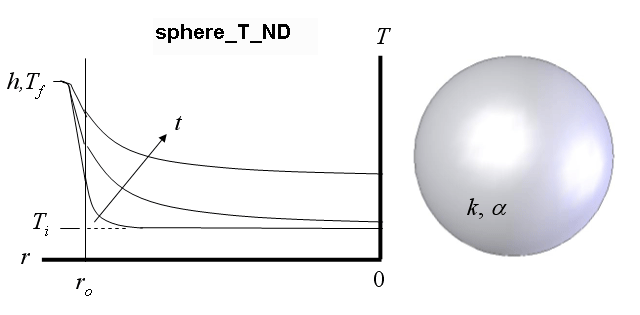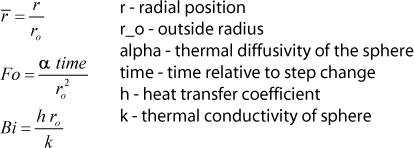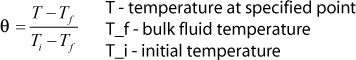Contents - Index

Sphere_T_NDFunction sphere_T_ND(r_bar, Fo, Bi) returns the non-dimensional temperature within a sphere whose outer surface (r=r_o) is exposed to convection. The supplied values, r_bar, Fo, Bi represent the dimensionless position, Fourier number and Biot number respectively.

Inputs:Output:Notes:
1.  Function sphere_T_ND is called by the function sphere_T to determine the non-dimensional temperature, theta, as described in Section 3.5.2 of Nellis and Klein.
2.  The solutions provided by EES are the 1st 20 terms of the infinite series solution
3.  The result for Fo<0.001 is determine by interpolation of the solution at Fo=0.001 and the known value (the initial condition) at Fo = 0.

Example:
r_bar=0.3
Fo=0.23
Bi=1.02
THETA=sphere_t_nd(r_bar, Fo, Bi)

{Solution:
THETA=0.6895}

Transient Conduction Index